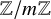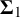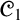### General logic (03Bxx)

• Article
• ##### Decidability of the class of all the rings$\mathbb {Z}/m\mathbb {Z}$: A problem of Ax
• Forum of Mathematics, Sigma, Volume 11

### Model theory (03Cxx)

• Article
• ##### Decidability of the class of all the rings$\mathbb {Z}/m\mathbb {Z}$: A problem of Ax
• Forum of Mathematics, Sigma, Volume 11

### Computability and recursion theory (03Dxx)

• Article
• ##### SOME CONSEQUENCES OF${\mathrm {TD}}$ AND${\mathrm {sTD}}$
• The Journal of Symbolic Logic, First View

### Set theory (03Exx)

• Article
• #####$\mathbf {\Sigma }_1$-definability at higher cardinals: Thin sets, almost disjoint families and long well-orders
• Forum of Mathematics, Sigma, Volume 11

• Article
• ##### USUBA’S PRINCIPLE$\mathrm {UB}_\lambda$ CAN FAIL AT SINGULAR CARDINALS
• The Journal of Symbolic Logic, First View

### Algebraic logic (03Gxx)

• Article
• ##### AN ABSTRACT ALGEBRAIC LOGIC STUDY OF DA COSTA’S LOGIC${\mathscr {C}}_1$ AND SOME OF ITS PARACONSISTENT EXTENSIONS
• Bulletin of Symbolic Logic, Volume 28, Issue 4